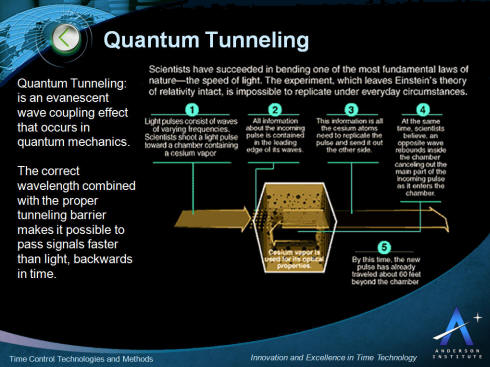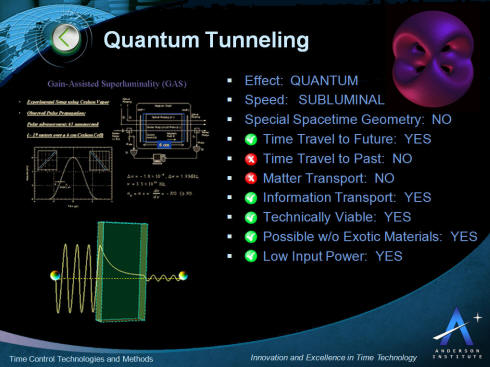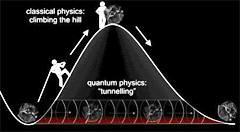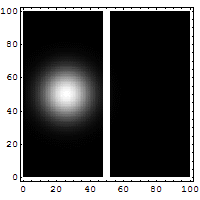Where history is becoming an experimental science Innovation and Excellence in Time Technology The Official Site of the World Encyclopedia of Time, the Time Shop, and the Time Research Association. Home  |  About Us  |  Educational Resources  |  Encyclopedia  |  The Time Shop  |  Time Research Association  |  Contact Us

## Quantum Tunneling

An Overview and Comparison by Dr. David Lewis Anderson

Quantum Tunneling is an evanescent wave coupling effect that occurs in quantum mechanics. The correct wavelength combined with the proper tunneling barrier makes it possible to pass signals faster than light, backwards in time.In the diagram above light pulses consisting of waves of various frequencies are shot toward a 10 centimeter chamber containing cesium vapor. All information about the incoming pulse is contained in the leading edge of its waves. This information is all the cesium atoms need to replicate the pulse and send it out the other side.

At the same time it is believed an opposite wave rebounds inside the chamber cancelling out the main part of the incoming pulse as it enters the chamber. By this time the new pulse, moving faster than the speed of light, has traveled about 60 feet beyond the chamber. Essentially the pulse has left the chamber before it finished entering, traveling backwards in time.

The key characteristics of the application of quantum tunneling for time control and time travel are presented in the picture below. This is followed by more detail describing the phenomenon below.Wave-mechanical tunneling (also called quantum-mechanical tunneling, quantum tunneling, and the tunnel effect) is an evanescent wave coupling effect that occurs in the context of quantum mechanics because the behavior of particles is governed by Schrödinger's wave-equation. All wave equations exhibit evanescent wave coupling effects if the conditions are right. Wave coupling effects mathematically equivalent to those called "tunneling" in quantum mechanics can occur with Maxwell's wave-equation (both with light and with microwaves), and with the common non-dispersive wave-equation often applied (for example) to waves on strings and to acoustics.

For these effects to occur there must be a situation where a thin region of "medium type 2" is sandwiched between two regions of "medium type 1", and the properties of these media have to be such that the wave equation has "traveling-wave" solutions in medium type 1, but "real exponential solutions" (rising and falling) in medium type 2. In optics, medium type 1 might be glass, medium type 2 might be vacuum. In quantum mechanics, in connection with motion of a particle, medium type 1 is a region of space where the particle total energy is greater than its potential energy, medium type 2 is a region of space (known as the "barrier") where the particle total energy is less than its potential energy.

If conditions are right, amplitude from a traveling wave, incident on medium type 2 from medium type 1, can "leak through" medium type 2 and emerge as a traveling wave in the second region of medium type 1 on the far side. If the second region of medium type 1 is not present, then the traveling wave incident on medium type 2 is totally reflected, although it does penetrate into medium type 2 to some extent. Depending on the wave equation being used, the leaked amplitude is interpreted physically as traveling energy or as a traveling particle, and, numerically, the ratio of the square of the leaked amplitude to the square of the incident amplitude gives the proportion of incident energy transmitted out the far side, or (in the case of the Schrödinger equation) the probability that the particle "tunnels" through the barrier.

### Quantum Tunneling IntroductionQuantum Tunneling
The scale on which these "tunneling-like phenomena" occur depends on the wavelength of the traveling wave. For electrons the thickness of "medium type 2" (called in this context "the tunneling barrier") is typically a few nanometers; for alpha-particles tunneling out of a nucleus the thickness is very much less; for the analogous phenomenon involving light the thickness is very much greater.

With Schrödinger's wave-equation, the characteristic that defines the two media discussed above is the kinetic energy of the particle if it is considered as an object that could be located at a point. In medium type 1 the kinetic energy would be positive, in medium type 2 the kinetic energy would be negative. There is no inconsistency in this, because particles cannot physically be located at a point: they are always spread out ("delocalized") to some extent, and the kinetic energy of the delocalized object is always positive.

What is true is that it is sometimes mathematically convenient to treat particles as behaving like points, particular in the context of Newton's Second Law and classical mechanics generally. In the past, people thought that the success of classical mechanics meant that particles could always and in all circumstances be treated as if they were located at points. But there never was any convincing experimental evidence that this was true when very small objects and very small distances are involved, and we now know that this viewpoint was mistaken. However, because it is still traditional to teach students early in their careers that particles behave like points, it sometimes comes as a big surprise for people to discover that it is well established that traveling physical particles always physically obey a wave-equation (even when it is convenient to use the mathematics of moving points). Clearly, a hypothetical classical point particle analyzed according to Newton's Laws could not enter a region where its kinetic energy would be negative. But, a real delocalized object, that obeys a wave-equation and always has positive kinetic energy, can leak through such a region if conditions are right. An approach to tunneling that avoids mention of the concept of "negative kinetic energy" is set out below in the section on "Schrödinger equation tunneling basics".Reflection and tunneling of an electron wave packet directed at a potential barrier. The bright spot moving to the left is the reflected part of the wave packet. A very dim spot can be seen moving to the right of the barrier. This is the small fraction of the wave packet that tunnels through the classically forbidden barrier. Also notice the interference fringes between the incoming and reflected waves.
An electron approaching a barrier has to be represented as a wave-train. This wave-train can sometimes be quite long – electrons in some materials can be 10 to 20 nm long. This makes animations difficult. If it were legitimate to represent the electron by a short wave-train, then tunneling could be represented as in the animation alongside.

It is sometimes said that tunneling occurs only in quantum mechanics. Unfortunately, this statement is a bit of linguistic conjuring trick. As indicated above, "tunneling-type" evanescent-wave phenomena occur in other contexts too. But, until recently, it has only been in quantum mechanics that evanescent wave coupling has been called "tunneling". (However, there is an increasing tendency to use the label "tunneling" in other contexts too, and the names "photon tunneling" and "acoustic tunneling" are now used in the research literature.)

With regards to the mathematics of tunneling, a special problem arises. For simple tunneling-barrier models, such as the rectangular barrier, the Schrödinger equation can be solved exactly to give the value of the tunneling probability (sometimes called the "transmission coefficient"). Calculations of this kind make the general physical nature of tunneling clear. One would also like to be able to calculate exact tunneling probabilities for barrier models that are physically more realistic. However, when appropriate mathematical descriptions of barriers are put into the Schrödinger equation, then the result is an awkward non-linear differential equation. Usually, the equation is of a type where it is known to be mathematically impossible in principle to solve the equation exactly in terms of the usual functions of mathematical physics, or in any other simple way. Mathematicians and mathematical physicists have been working on this problem since at least 1813, and have been able to develop special methods for solving equations of this kind approximately. In physics these are known as "semi-classical" or "quasi-classical" methods. A common semi-classical method is the so-called WKB approximation (also known as the "JWKB approximation"). The first known attempt to use such methods to solve a tunneling problem in physics was made in 1928, in the context of field electron emission. It is sometimes considered that the first people to get the mathematics of applying this kind of approximation to tunneling fully correct (and to give reasonable mathematical proof that they had done so) were N. Fröman and P.O. Fröman, in 1965. Their complex ideas have not yet made it into theoretical-physics textbooks, which tend to give simpler (but slightly more approximate) versions of the theory. An outline of one particular semi-classical method is given below.Three notes may be helpful. In general, students taking physics courses in quantum mechanics are presented with problems (such as the quantum mechanics of the hydrogen atom) for which exact mathematical solutions to the Schrödinger equation exist. Tunneling through a realistic barrier is a reasonably basic physical phenomenon. So it is sometimes the first problem that students encounter where it is mathematically impossible in principle to solve the Schrödinger equation exactly in any simple way. Thus, it may also be the first occasion on which they encounter the "semi-classical-method" mathematics needed to solve the Schrödinger equation approximately for such problems. Not surprisingly, this mathematics is likely to be unfamiliar, and may feel "odd". Unfortunately, it also comes in several different variants, which doesn't help.

Also, some accounts of tunneling seem to be written from a philosophical viewpoint that a particle is "really" point-like, and just has wave-like behavior. There is very little experimental evidence to support this viewpoint. A preferable philosophical viewpoint is that the particle is "really" delocalized and wave-like, and always exhibits wave-like behavior, but that in some circumstances it is convenient to use the mathematics of moving points to describe its motion. This second viewpoint is used in this section. The precise nature of this wave-like behavior is, however, a much deeper matter, beyond the scope of this article on tunneling.

Although the phenomenon under discussion here is usually called "quantum tunneling" or "quantum-mechanical tunneling", it is the wave-like aspects of particle behavior that are important in tunneling theory, rather than effects relating to the quantization of the particle's energy states. For this reason, some writers prefer to call the phenomenon "wave-mechanical tunneling.

### History

By 1928, George Gamow had solved the theory of the alpha decay of a nucleus via tunneling. Classically, the particle is confined to the nucleus because of the high energy requirement to escape the very strong potential. Under this system, it takes an enormous amount of energy to pull apart the nucleus. In quantum mechanics, however, there is a probability the particle can tunnel through the potential and escape. Gamow solved a model potential for the nucleus and derived a relationship between the half-life of the particle and the energy of the emission.

Alpha decay via tunneling was also solved concurrently by Ronald Gurney and Edward Condon. Shortly thereafter, both groups considered whether particles could also tunnel into the nucleus.

After attending a seminar by Gamow, Max Born recognized the generality of quantum-mechanical tunneling. He realized that the tunneling phenomenon was not restricted to nuclear physics, but was a general result of quantum mechanics that applies to many different systems. Today the theory of tunneling is even applied to the early cosmology of the universe.

Quantum tunneling was later applied to other situations, such as the cold emission of electrons, and perhaps most importantly semiconductor and superconductor physics. Phenomena such as field emission, important to flash memory, are explained by quantum tunneling. Tunneling is a source of major current leakage in Very-large-scale integration (VLSI) electronics, and results in the substantial power drain and heating effects that plague high-speed and mobile technology.

Another major application is in electron-tunneling microscopes which can resolve objects that are too small to see using conventional microscopes. Electron tunneling microscopes overcome the limiting effects of conventional microscopes (optical aberrations, wavelength limitations) by scanning the surface of an object with tunneling electrons.

Quantum tunneling has been shown to be a mechanism used by enzymes to enhance reaction rates. It has been demonstrated that enzymes use tunneling to transfer both electrons and nuclei such as hydrogen and deuterium. It has even been shown, in the enzyme glucose oxidase, that oxygen nuclei can tunnel under physiological conditions.